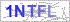## JavaScript继承方式详解

标签：JavaScript,js继承,js原型链
修改 | 阅读（431）| 评论（0）

js继承的概念

js里常用的如下两种继承方式：

js可以通过构造函数和原型的方式模拟实现类的功能。 另外，js类式继承的实现也是依靠原型链来实现的。

```function Super() {
this.colors = ["red", "blue"];
}
function Sub() {
Super.call(this);
}```

```function Parent() {
this.name = 'mike';
}
function Child() {
this.age = 12;
}
Child.prototype = new Parent(); //Child继承Parent，通过原型，形成链条
var test = new Child();
//继续原型链继承
function Brother() {   //brother构造
this.weight = 60;
}
Brother.prototype = new Child();//继续原型链继承
var brother = new Brother();

```alert(brother instanceof Object)//true

```function Parent(age) {
this.name = ['mike', 'jack', 'smith'];
this.age = age;
}
function Child(age) {
Parent.call(this, age);
}
var test = new Child(21);
test.name.push('bill');

```function Parent(age) {
this.name = ['mike', 'jack', 'smith'];
this.age = age;
}
Parent.prototype.run = function () {
return this.name + ' are both' + this.age;
};
function Child(age) {
Parent.call(this, age);
}
Child.prototype = new Parent();
var test = new Parent(21);//写new Child(21)也行

```function obj(o) {
function F() { }
F.prototype = o;
return new F();
}
var box = {
name: 'trigkit4',
arr: ['brother', 'sister', 'baba']
};
var b1 = obj(box);
b1.name = 'mike';
b1.arr.push('parents');
var b2 = obj(box);

```function create(o){
var f= obj(o);
f.run = function () {
return this.arr;//同样，会共享引用
};
return f;
}```

```function Parent(name){
this.name = name;
this.arr = ['哥哥','妹妹','父母'];
}
Parent.prototype.run = function () {
return this.name;
};
function Child(name,age){
Parent.call(this, age);//第二次调用
this.age = age;
}
Child.prototype = new Parent();//第一次调用```

```function obj(o){
function F(){}
F.prototype = o;
return new F();
}
function create(parent,test){
var f = obj(parent.prototype);//创建对象
f.constructor = test;//增强对象
}
function Parent(name) {
this.name = name;
this.arr = ['brother', 'sister', 'parents'];
}
Parent.prototype.run = function () {
return this.name;
};
function Child(name, age) {
Parent.call(this, name);
this.age = age;
}
inheritPrototype(Parent, Child);//通过这里实现继承
var test = new Child('trigkit4', 21);
test.arr.push('nephew');
var test2 = new Child('jack', 22);

call和apply

```// 定义一个全局函数
function foo() {
console.log(this.fruit);
}
// 定义一个全局变量
var fruit = "apple";
// 自定义一个对象
var pack = {
fruit: "orange"
};
// 等价于window.foo();
foo.apply(window);
// "apple",此时this等于window
// 此时foo中的this === pack
foo.apply(pack);    // "orange"```

QQ登录(无需注册直接登录可进行回复)